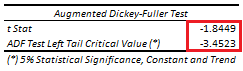# Stationarity: Augmented Dickey-Fuller Test

Last Update: May 15, 2022

Stationarity is when time series statistical properties do not change over time. First order stationarity is when time series mean does not change over time. This can be tested through augmented Dickey-Fuller test  which evaluates whether time series has a unit root. If time series does not have a unit root, then it is first order stationary.

As example, we can delimit univariate time series  into training range  for model fitting and testing range  for model forecasting.

Then we can do training range augmented Dickey-Fuller test regression with formula . Training range augmented Dickey-Fuller test regression fitted values differences  are the estimated  values differences with formula ,  are previous period values, is deterministic trend variable and  are previous  period  values differences. Notice that we have to test whether constant, deterministic trend variable and which training range values differences number of lags are needed within augmented Dickey-Fuller test.

Next, we do t-test with individual null hypothesis that training range previous period  values coefficient is equal to zero or that time series has a unit root with formula . If individual null hypothesis is rejected, then training range time series does not have a unit root and it is first order trend stationary or assumed with a constant mean around a deterministic trend when including  lags of training range values differences in test. Notice that a unit root biases the estimation of coefficient downward and augmented Dickey-Fuller test specific critical values  would be needed for t-test.

Also, notice that we can alternatively do an F-test with joint null hypothesis that training range previous period values and deterministic trend variable coefficients are equal to zero or that time series has a unit root and/or does not have a deterministic trend with formula . If joint null hypothesis is rejected, then training range time series does not have a unit root and/or has a deterministic trend and it is first order trend stationary or assumed with a constant mean around a deterministic trend when including  lags of training range values differences in test. Additionally, notice that joint null hypothesis test  would require additional individual coefficients tests to evaluate training range time series does not have a unit root and/or has a deterministic trend. Furthermore, notice that a unit root biases the estimation of coefficient downward and augmented Dickey-Fuller test specific critical values would be needed for F-test.

Below, we find example of training range augmented Dickey-Fuller test with constant, deterministic trend variable and twelve lags of training range values differences using airline passengers data . Training range as first ten years and testing range as last two years of data.Table 1. Microsoft Excel® training range augmented Dickey-Fuller test with constant, deterministic trend variable and twelve lags of training range values differences using airline passengers data.

 Dickey, D. A.; Fuller, W. A. (1979). “Distribution of the Estimators for Autoregressive Time Series with a Unit Root”. Journal of the American Statistical Association. 74 (366): 427–431.

 Fuller, W. A. (1976). “Introduction to Statistical Time Series”. New York: John Wiley and Sons.

 Data Description: Monthly international airline passenger numbers in thousands from 1949 to 1960.

Original Source: Box, G. E. P., Jenkins, G. M. and Reinsel, G. C. (1976). “Time Series Analysis, Forecasting and Control”. Third Edition. Holden-Day. Series G.

Source: datasets R Package AirPassengers Object. R Core Team (2021). “R: A language and environment for statistical computing”. R Foundation for Statistical Computing, Vienna, Austria.

+